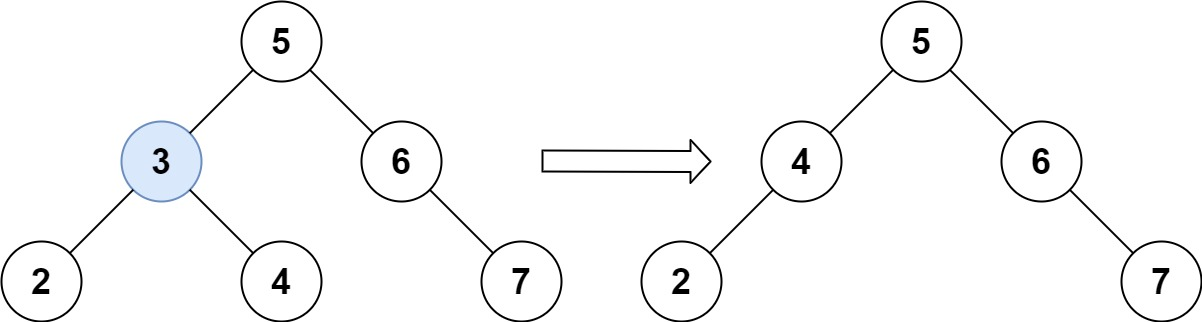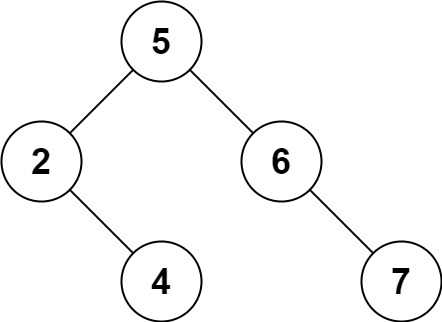### LeetCode• ㊗️
• 大家
• offer
• 多多！

## Problem

Given a root node reference of a BST and a key, delete the node with the given key in the BST. Return the root node reference (possibly updated) of the BST.

Basically, the deletion can be divided into two stages:

Search for a node to remove. If the node is found, delete the node. Follow up: Can you solve it with time complexity O(height of tree)?

Example 1:Input: root = [5,3,6,2,4,null,7], key = 3
Output: [5,4,6,2,null,null,7]
Explanation: Given key to delete is 3. So we find the node with value 3 and delete it.
One valid answer is [5,4,6,2,null,null,7], shown in the above BST.
Please notice that another valid answer is [5,2,6,null,4,null,7] and it's also accepted.Example 2:

Input: root = [5,3,6,2,4,null,7], key = 0
Output: [5,3,6,2,4,null,7]
Explanation: The tree does not contain a node with value = 0.


Example 3:

Input: root = [], key = 0
Output: []


Constraints:

• The number of nodes in the tree is in the range [0, 104].
• -10^5 <= Node.val <= 10^5
• Each node has a unique value.
• root is a valid binary search tree.
• -10^5 <= key <= 10^5

## Code

class Solution {
public TreeNode deleteNode(TreeNode root, int key) {
if(root == null) return root;

// 三种情况
if(key > root.val){
root.right = deleteNode(root.right, key);
} else if (key < root.val){
root.left = deleteNode(root.left, key);
// 需要删除root
} else {
// 三种情况
if(root.left == null) {
return root.right;
} else if (root.right == null) {
return root.left;
} else{
// 找到右子树的最小值
TreeNode min = root.right;
while(min.left != null){
min = min.left;
}

// 把min节点的值赋值给root
root.val = min.val;
// 删除右子树中的min节点
root.right = deleteNode(root.right, min.val);
}
}

return root;
}
}